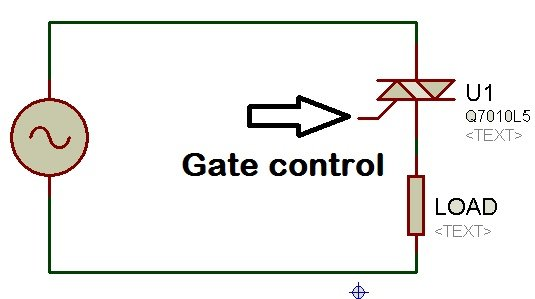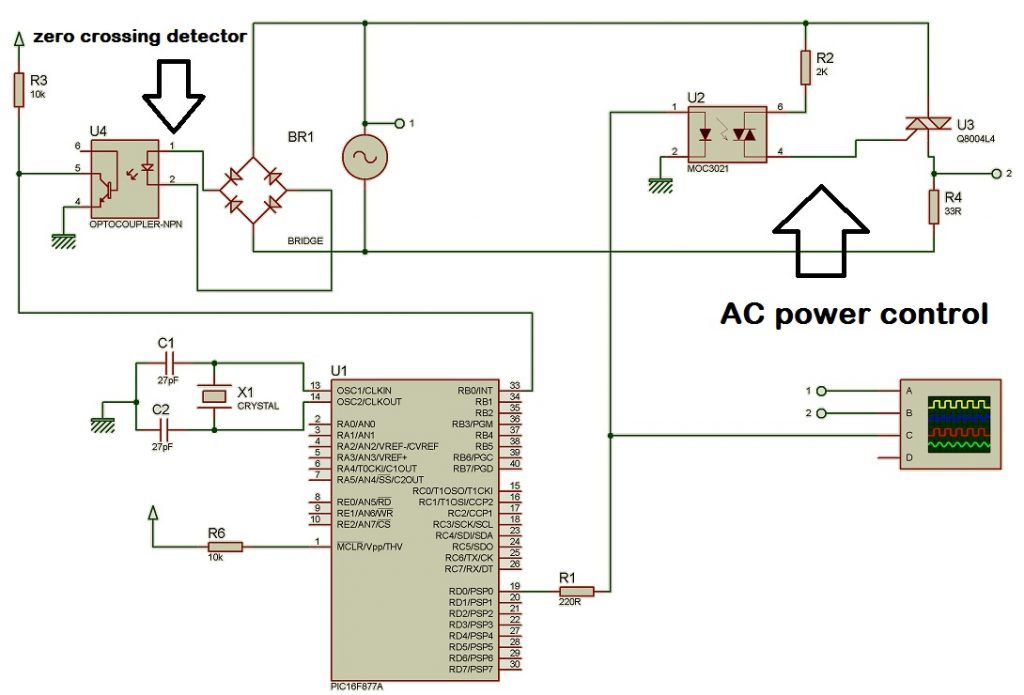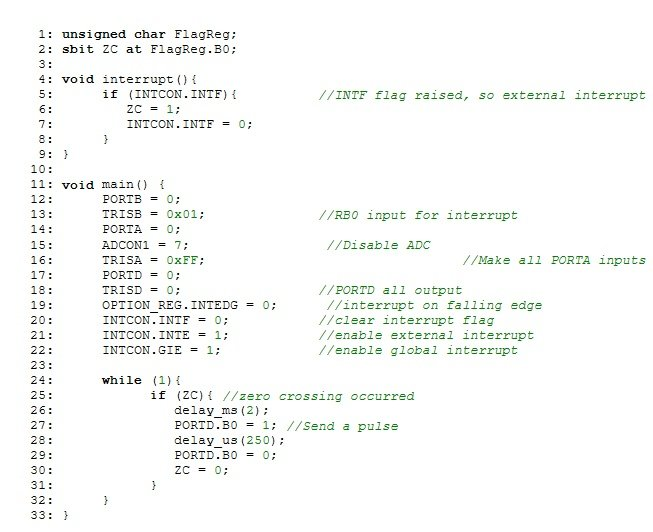# AC power control with thyristor using pic microcontroller

AC power control with thyristor using pic microcontroller project is designed to control AC power flow across load. This project is designed using PIC16F877A microcontroller and Zero crossing detection circuit. Phase angle control method is used for AC power control with thyristor. Thyristor is used as a switch to control flow of power. PIC16F877A microcontroller is used to detect zero crossing and to generate pulse signals at a specific angle for thyristor.

### AC power control with thyristor

Lets start with basic understanding of AC power control with thyritor.  For example if a load is connected to AC power supply through a switch as shown in figure below. Triac is used as a switch. Triac is a kind of thyrsitor. In triac two back to back thyristors are connected to each other.The AC power flow to load can be controlled by controlling rms value of voltage appearing across load as shown in above figure. This method is also called AC voltage controller method. In AC voltage controller, rms value of voltage appearing across load is controlled by varying the firing angle of triac gate signal as shown in figure above. As you know power in AC circuits power ( Active power) is calculated by using following formula:

P = V × I × cos(θ) Where  cos(θ) is power factor

To make this project more easier we used only resistive load. By making little bit changes in code, you can also use it with inductive loads. In resistive loads power factor is always unity. So  cos(θ) = 1 and above formula becomes:

P = V × I = V × V/ R = V²/R

P= V² /R

So according to above formula, power across load is directly proportional to square of voltage. By controlling voltage across load, power can also be calculated. As I have mentioned earlier, AC voltage controllers are used to control rms value of voltage across loads. AC voltage controller circuits have many applications in industry like tap changers in transformers, induction heating, speed control of induction motor and  lights control.

### Types of AC voltage controller

Two types of AC voltage controller are used for AC power control with thyristor. Breif explanation of both of them is given below:

• Pulse skipping modulation control : In this method load is connected to ac power supply for few cycles and then supply is turned off for few cycles. AC power supply is controlled by controlling firing angle of thyristor.
• Phase angle control : In phase angle control method, loads are connected across AC supply for specific portion of positive and negative cycle. Specific portion depends on timing of thristor firing angle.

In AC power control with thyristor project, phase angle control method is used to control flow of power across load by controlling voltage.

### Circuit diagram of AC power control with thyristor

Circuit diagram of AC power control with thyristor using pic microcontroller.  I have also made necessary comments in figure.PIC16F877A microcontroller is used to detect zero crossing of AC voltage and PIC16F877A microcontroller generate pulse for triac a specific delay after zero crossing detection. I have also posted a article on zero crossing detection using PIC16F877A microcontroller. For more understanding about zero crossing detection check following article:

Zero crossing detection using pic microcontroller

In this project I have used a delay of 2ms. What its means? Its means microcontroller will generate a pulse after zero crossing detection with 2ms delay. You can adjust the delay either by manually entering its value in code or you can also use variable resistor to adjust value of delay  by using analog to digital converter of PIC16F877A microcontroller.There is not too much science involve involved in above circuit diagram of ac power control with thyrsitor.  First of all microcontroller checks zero crossing detection and then fired triac angle with 2ms delay after zero crossing detection.

### AC power control with thyristor program

Code for AC power controller project is written using Mikro C pro compiler. Simply put this code in mikro C compiler after creating new project. 11.059MHz Crsytal is used for this project.Necessary comments are also made in above code. Code for AC power control is self explanatory, but if you still feel any issue, feel free to comment on this post with your issues.

### 31 thoughts on “AC power control with thyristor using pic microcontroller”

1. I want to learn how to program micro controller to be used for a DC/AC inverter. I normally use PWM IC before (SG3524). What are the requirement I need. Thank you sir. Abiola Sunday Adewale.

• Sir,
I want thyristor control using two pushbuttons. One for phase increment and one for decrement.
Also angle is stored in eeprom. So that when power up the system, with previous phase angle SCR is fired.
Hope you will do needful.
Krunal Thummar,
Rajkot (Gujarat-India)

2. Hai sir. Thanks for your information regarding ac power control. I am doing a project on speed control of induction motor using thyristor and 8051 microcontroller. I am stuck up with how to apply control pulse with a push button or switch. Please explain me about how to control this firing pulse externally

• Can u upload the proteus and hex file of this circuit.

• Hey, have you finished you project.
Please let me know because I am also doing the same project

3. hello bilal. good explaination.
since you have used a 2 ms delay after getting zero crossing sensing, does this means that for 50 hz supply, this will deliver 80% power to the load. And similarly to drive the load at 20 % , i will need to introduce a delay of 8 ms.
Thanks

• Where is the code sir??

4. How to make circuit diagram of AC power control with thyristor

6. Hi, I need to drive a output load of 300watts between 0 to 65Vac, i am using your circuit and code as well, but my out put volatge is always between 111vac to 118vac. Please suggest what should be the triac firing angle duration.

7. hi, i want control the speed of ac induction motor with 89S52 controller ,am getting only high with the circuit of triac and am giving external inetrrupt to controller to change the speed but when am giving interrupt completly motor is going to off state..please suggest any need ful information .

8. can we use MC2TE for ZCD in this circuit..?? and suggest me any circuit diagram if any have..???

phase angle of scr using pic microcontrol

10. Hi,
I wanna ask you something. Can i know how the simulation of this circuit work and where is the input area of this project. Is it on zero detector ? If yes why the altenator is on the middle of the circuit. Hope you can answer. Thanks

11. Why the ground is not connected to bridge rectifier

12. Hi,
I hope you reply it as soon as possible.

13. i want to working of programmable ac power contol using thyristor based on firing angle principle

14. i am not getting any out put in proteus for the same code

15. Do you have this, but for a three phase control?

16. Please tell me how to buy this code from you. I need it so much!!

17. ​sir,

cud u please let me know how triac will be used in ac for speed control of fan and on/off of light without using opto isolator/opto driver.

tnx

sa